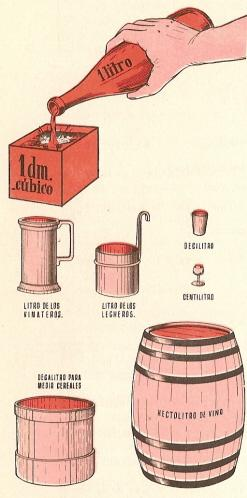Metric units of capacity to printName: ______________________________________  Subject: _______________________ Date: _______

Write on the right side what is missing.

1. The liter

The units of capacity are used to measure liquids. The fundamental unit for measuring capacity is the liter which is equal to a cubic decimeter. In the picture, we see that the fluid of a 1 liter container fits in a box that is one decimeter on each side.

The abbreviation for liter is l.

2. Multiples of the liter

These are:

1 decaliter is equal to 10 liters: 1 dal = 10 l.
1 hectoliter is equal to 100 liters: 1 hl = 100 l.
1 kiloliter is equal to 1000 liters: 1 kl = 1000 l.
1 myrialiter is equal to 10000 liters: 1 myl = 10000 l.

 7 dal = 6 kl = 4 mal = 2 dal = 9 hl = 3kl = 5 mal =3. - Submultiples of the liter

These are:

1 deciliter is equal to 0.1 liters: 1 dl = 0.1 l. 1 liter is equal to 10 deciliters.
1 centiliter is equal to 0.01 liters: 1 cl = 0.01 l. 1 liter is equal to 100 centiliters.
1 milliliter is equal to 0.001 liters: 1 ml = 0.001 l. 1 liter is equal to 1000 milliliters.

 4 dl = 1 ml = 3 dl = 6 ml = 5 cl = 3 cl =

4. Conversion of unit

Each metric unit of capacity is 10 times greater than the immediate lower and 10 times less than the immediate superior.

To convert from kl to hl, we multiply by 10 or move the decimal point one place to the right.
Examples: 18 kl = 180 hl: 17,35 hl =173,5 dal = 1735 l.

To convert from l to dal, we divide by 10 or we move the decimal point one place to the left.
Examples: 80 l = 8 dal;  1375,2 l = 137,52 dal = 13,752 hl = 1,3752 kl.

 7 dal = 8,5 hl = 1,35 kl = 250 dl = 3134 cl = 305 ml =

5. Complex and non-complex numbers

The pot capacity is 2 l, 7 dl and 5 cl. This number, forming by different units, is called complex number. If we add 2 l + (7 dl = 0.7 l) + 5 cl = 0.05 l) = 2.75 l. The pot capacity is 2. 75 l. This is a non-complex number, because it is expressed in a single unit of measurement.

To convert from complex number to non-complex number of lower order, we must write the numbers that represent the units of the various orders from left to right, and one after the other. We have to start with the greatest order. If it lacks some order, we must put a zero in the appropriate place.

Example: 7 kl, 6 dal, 3 l and 2 dl can be written as: 70632 dl = 7063.2 l.

 4 mal, 7 hl y 2 cl = 5 kl, 3 dal y 2 dl = 8 hl, 2 l, 3 dl y 7 cl = 3 dal, 4 l y 7 ml = 8 dl, 7 cl y 4 ml =

6. Converting from non-complex number (75 l) to complex number (7 dal and 5 l)

To convert from non-complex number to complex number, we have to take into account that the number of units has the same order as the non-complex, the tens of the immediate superior order and so on. If there are zeros in the number, we avoid the zero and skip the order that corresponds to it.

Example: 5203.65 l can be written as: 5 kl, 2 hl, 3 l, 6 dl and 5 cl.

Answers in non-complex number:

 7,08 l = 304 l = 80,9 hl = 0,65 kl = 602 dl =

| Educational applications | Mathematics |
In Spanish  | Interactive

«Arturo Ramo GarcĒa.-Record of intellectual property of Teruel (Spain) No 141, of 29-IX-1999
Plaza Playa de Aro, 3, 1║ DO 44002-TERUEL